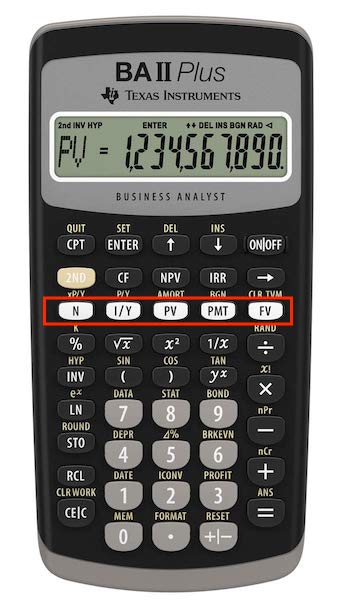# Calculate Price of a Bond Using Texas Instruments BA II+ Calculator

This article demonstrates the basic functionality of the Texas Instruments BA II Plus Financial Calculator. It teaches you how to use the calculator to calculate the price of a bond. What is the price of a 10-year $1,000 face value bond with a coupon rate of 6.0% that pays annually, if the yield is 8.0%? Let’s first understand the characteristics of the bond. The bond has a maturity of 10 years. It has an annual coupon rate of 6%, and the coupon is paid semiannually. So, the investor received a coupon payment of$30 every 6 months. The bond’s yield (yield-to-maturity) is 8%.

Using the bond price formula, the value of the bond is $864.10. Let’s calculate this using the BA II Plus Calculator. A good thing about this calculator is that it puts the five variables in one row. Out of these 5 variables, we have the 4 we know and calculate the fifth. The five variables are highlighted below:These keys are labeled as the time value of money variables. In terms of the bond, they will be as follows: N=Number of Period I/Y=Yield per Period PV=Discounted Bond Price PMT=Coupon per Period FV=Face Value We have N, I/Y, PMT, and FV, and we are calculating for PV. N = 20 (102) I/Y = 4% (8/2) PMT = 30 (10000.06/2) FV = 1000 We are solving for PV. To enter the above values in the calculator, you first enter the value and then press the variable key. For example, if you’re entering the period, you first enter 20 in the calculator and then press the N key. This will commit the value 20 to variable N. Do the same for the other variables. Once you have entered N, I/Y, PMT, and FV, it's time to calculate PV. To do so, press the CPT key (top left key), and then press the PV key. The screen will now show the price of the bond, which should be -$864.10. The calculator shows a negative value because it’s an outflow of cash to buy the bond. There is a cash outflow of $864.10 and at the end of 10 years, there will be a cash inflow of$1000. In between, there is a cash inflow of $30 every 6 months. What will happen if the bond was paying coupons annually instead of semi-annually? Try calculating the price of the bond with annual payments using your calculator. The price of the bond should be$865.80.

Note that from these 5 variables, you can input any four and calculate the fifth one for the bond.